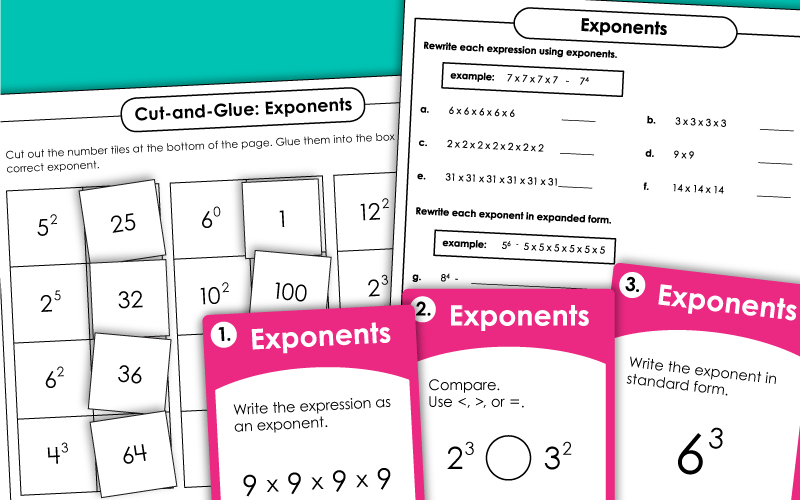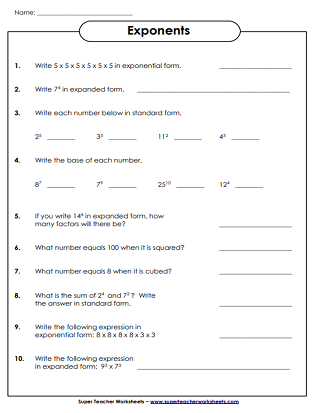# Exponents

Use these worksheets and task cards to help students learn about exponents. Most worksheets on this page align with Common Core Standard 6.EE.1.## Exponents w/ WholeNumbers as a Base

Exponents  FREE
Write the exponents as numbers in expanded form and standard form.
This file contains 30 task cards with exponent problems on them.
Compare the values of the exponents using the greater and less than symbols.
Here's another fortune teller game that requires players to find the squares of certain numbers. Color, cut, fold, and play!
Cut out the number tiles at the bottom of the page. Glue each number next to the equivalent exponent.

## Exponentsw/ Decimal Bases

Evaluate the expressions. Find the values of the exponents with decimal bases.

Scientific Notation

Practice writing numbers in scientific notation.

Squared Numbers and Square Roots

Review squared numbers and square roots with these printables.

Place Value Worksheets

These worksheets can be used for teaching comparing numbers, ordering, and expanded form.

## Pictures of Our WorksheetsMy Account
Site Information